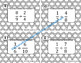# Dividing Fractions by Fractions Task CardsSubject
Resource Type
File Type

PDF

(448 KB|7 pages)
Product Rating
Standards
• Product Description
• StandardsNEW

Dividing Fractions by Fractions Task Cards

Included:

-20 cards included

Apply and extend previous understandings of multiplication and division and of fractions to multiply and divide rational numbers.
Fluently add, subtract, multiply, and divide multi-digit decimals using the standard algorithm for each operation.
Fluently divide multi-digit numbers using the standard algorithm.
Interpret and compute quotients of fractions, and solve word problems involving division of fractions by fractions, e.g., by using visual fraction models and equations to represent the problem. For example, create a story context for (2/3) ÷ (3/4) and use a visual fraction model to show the quotient; use the relationship between multiplication and division to explain that (2/3) ÷ (3/4) = 8/9 because 3/4 of 8/9 is 2/3. (In general, (𝘢/𝘣) ÷ (𝘤/𝘥) = 𝘢𝘥/𝘣𝘤.) How much chocolate will each person get if 3 people share 1/2 lb of chocolate equally? How many 3/4-cup servings are in 2/3 of a cup of yogurt? How wide is a rectangular strip of land with length 3/4 mi and area 1/2 square mi?
Interpret a fraction as division of the numerator by the denominator (𝘢/𝘣 = 𝘢 ÷ 𝘣). Solve word problems involving division of whole numbers leading to answers in the form of fractions or mixed numbers, e.g., by using visual fraction models or equations to represent the problem. For example, interpret 3/4 as the result of dividing 3 by 4, noting that 3/4 multiplied by 4 equals 3, and that when 3 wholes are shared equally among 4 people each person has a share of size 3/4. If 9 people want to share a 50-pound sack of rice equally by weight, how many pounds of rice should each person get? Between what two whole numbers does your answer lie?
Total Pages
7 pages
Included
Teaching Duration
N/A
Report this Resource to TpT
Reported resources will be reviewed by our team. Report this resource to let us know if this resource violates TpT’s content guidelines.